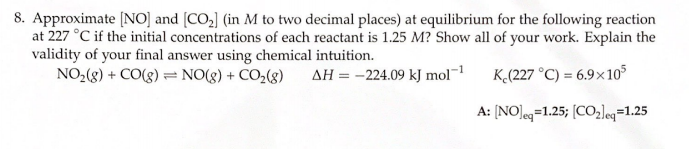# 8. Approximate [NO] and [CO2] (in M to two decimal places) at equilibrium for the following reaction at 227 degrees C if the initial concentrations of each reactant is 1.25 M? Show all of your work. Explain the validity of your final answer using chemical intuition. NO2(g) + CO(g) ⇌ NO(g) + CO2(g) ΔH = -224.09 kJ mol^-1 Kc(227 degrees C) = 6.9 x 10^5